3.1 Schrödinger-Poisson Solver

All NBTI models share the same challenge, namely to explain the correct field acceleration and temperature activation of the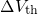degradation. These experimentally observed dependences must be traced back to the physics in a MOSFET. The most frequently used charge trapping models require the band diagram, the electric field across the insulator, and the spatial distribution of the inversion charge carriers. This information can easily be computed via a Poisson-solver (P-solver) or a Schrödinger-Poisson solver (SP-solver)  if quantum mechanics are assumed to play a crucial role.

The electrostatics within a MOS device are described by the Poisson equation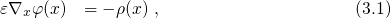where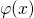denotes the electrical potential. The charge density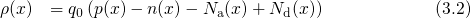is decomposed into the charge carrier densities of the electrons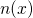and holes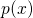and the ionized dopant concentration of acceptor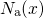and donatoratoms. The charge carrier densities at the point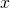are expressed as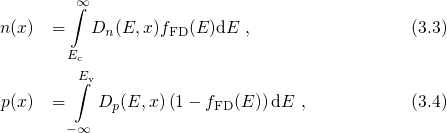where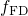stands for the Fermi-Dirac distribution, which determines the occupation of the conduction and valence band states and is given by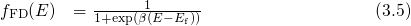Its validity rests upon thermal equilibrium between the charge carriers in a specific region of the MOS device. This assumption is well justified when no voltage is applied between source and drain of a MOSFET during NBTI stress. In the parabolic band approximation the electron () and hole (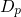) DOS in equation (3.3) and (3.4) are defined by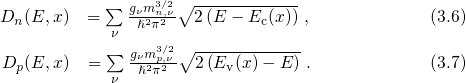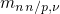is the electron/hole effective mass with a degeneracy of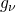, where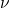denotes the valley index.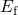,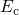, and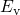stand for the Fermi energy, the conduction, and the valence band edge, respectively. The electrostatic potentialenters the calculation of the conduction (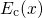) and the valence (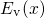) band edge as follows: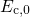and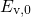denote the conduction and the valence band edge energy in the flat band case, respectively. Due to the mutual dependence between, on the one hand, and the carrier densitiesand, on the other hand, the equations (3.1)-(3.9) must be solved self-consistently. This has been achieved by a P-solver, whose functionality relies on a numerical iteration scheme, visualized in Fig. 3.1.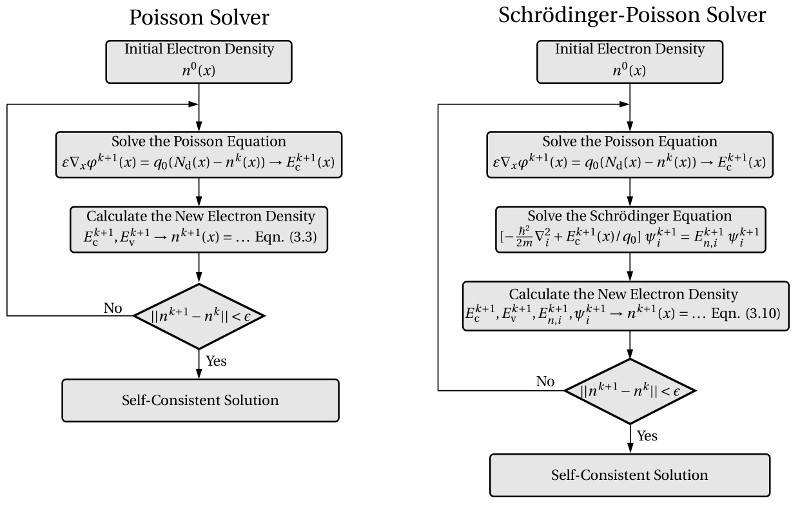Figure 3.1: A flow chart diagram of the Newton iteration scheme used in the Poisson (left) and the Schrödinger-Poisson solver (right). The procedure is only discussed for electrons in the conduction band for simplicity but it also holds true for holes in the valence band using analogous formulas. In both iteration schemes, the old electron density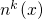enters the Poisson equation and a new electrical potential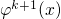is obtained, which is then required to calculate the new electron density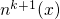. In a Poisson solver the new electron densityis evaluated using the semi-classical formula (3.3). By contrast, in the Schrödinger-Poisson solver the bound states must be determined, which are then taken to evaluate the new electron densityaccording to equation (3.10). Using the newly computed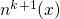, the iteration loop is continued at the Poisson step until the update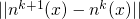falls below a certain limit.

More realistic simulations must account for the quantum confinement of the charge carriers in the inversion layer. This effect arises from the band bending, which forms a potential well for one type of charge carriers. As described in Section2.1, this well enters as the potential in the one-dimensional Schrödinger equation, whose solution consists of the single quasi-bound states of electrons and holes. These states are required for the calculation of the carrier densities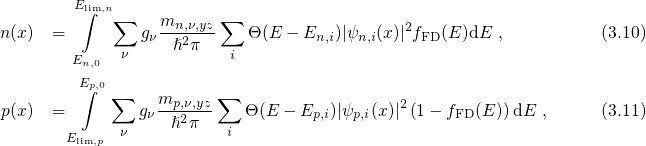where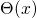stands for the Heaviside step function defined by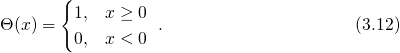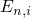denotes the energy of the electron quasi-bound stateand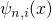is the corresponding channel wavefunction. The single subbands of the quasi-bound states add up to the electron DOS within the potential well, which is formed by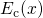and limited by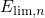. Outside this region, the charge carriers are calculated according to equations (3.6) for the free states. Similarly, the hole quasi-bound states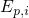with the channel wavefunction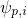form subbands within the energy range between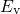and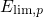. For the calculation of the band diagram, the Poisson equation and the Schrödinger equation must be solved self-consistently since they are coupled through the electrostatic potentialand the charge carrier concentrations,and. In a SP-solver, this system of coupled equations is treated using a self-consistent iteration method, outlined in Fig. 3.1. Throughout this thesis, the Vienna Schrödinger Poisson (VSP) solver  has been applied for the calculation of the band diagram and the charge carrier concentrations.